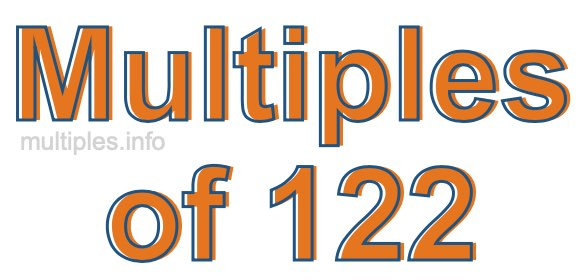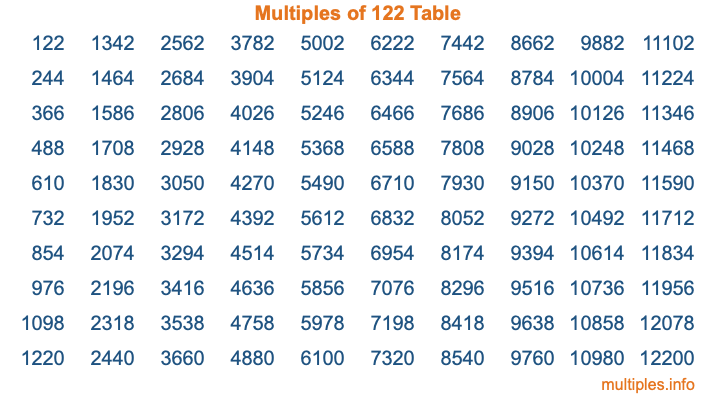Multiples of 122Welcome to the Multiples of 122 page. Here we will first teach you everything you will ever need to know about the multiples of 122, and then give you a study guide summary of everything we taught you to make sure you remember it all. Use this page to look up facts and learn information about the multiples of 122. This page will make you a multiples of one hundred twenty-two expert!

Definition of Multiples of 122
Multiples of 122 are all the numbers that when divided by 122 equal an integer. Each of the multiples of 122 are called a multiple. A multiple of 122 is created by multiplying 122 by an integer.

Therefore, to create a list of multiples of 122, you start with 1 multiplied by 122, then 2 multiplied by 122, then 3 multiplied by 122, and so on for as long as you want. Thus, the list of the first five multiples of 122 is 122, 244, 366, 488, and 610. To see a larger list of multiples of 122, see the printable image of Multiples of 122 further down on this page. We also have a category where you can choose any nth multiple of 122.

Multiples of 122 Checker
The Multiples of 122 Checker below checks to see if any number of your choice is a multiple of 122. In other words, it checks to see if there is any number (integer) that when multiplied by 122 will equal your number. To do that, we divide your number by 122. If the the quotient is an integer, then your number is a multiple of 122.

Is  a multiple of 122?

Least Common Multiple of 122 and ...
A Least Common Multiple (LCM) is the lowest multiple that two or more numbers have in common. This is also called the smallest common multiple or lowest common multiple and is useful to know when you are adding our subtracting fractions. Enter one or more numbers below (122 is already entered) to find the LCM.

Check out our LCM Calculator if you need more details about the Least Common Multiple or if you need the LCM for different numbers for adding and subtraction fractions.

nth Multiple of 122
As we stated above, 122 is the first multiple of 122, 244 is the second multiple of 122, 366 is the third multiple of 122, and so on. Enter a number below to find the nth multiple of 122.

th multiple of 122

Multiples of 122 vs Factors of 122
122 is a multiple of 122 and a factor of 122, but that is where the similarities end. All postive multiples of 122 are 122 or greater than 122. All positive factors of 122 are 122 or less than 122.

Below is the beginning list of multiples of 122 and the factors of 122 so you can compare:

Multiples of 122: 122, 244, 366, 488, 610, etc.

Factors of 122: 1, 2, 61, 122

As you can see, the multiples of 122 are all the numbers that you can divide by 122 to get a whole number. The factors of 122, on the other hand, are all the whole numbers that you can multiply by another whole number to get 122.

It's also interesting to note that if a number (x) is a factor of 122, then 122 will also be a multiple of that number (x).

Multiples of 122 vs Divisors of 122
The divisors of 122 are all the integers that 122 can be divided by evenly. Below is a list of the divisors of 122.

Divisors of 122: 1, 2, 61, 122

The interesting thing to note here is that if you take any multiple of 122 and divide it by a divisor of 122, you will see that the quotient is an integer.

Multiples of 122 Table
Below is an image of the first 100 multiples of 122 in a table. The table is in chronological order, column by column. The first column has the first ten multiples of 122, the second column has the next ten multiples of 122, and so on.The Multiples of 122 Table is also referred to as the 122 Times Table or Times Table of 122. You are welcome to print out our table for your studies.

Negative Multiples of 122
Although not often discussed or needed in math, it is worth mentioning that you can make a list of negative multiples of 122 by multiplying 122 by -1, then by -2, then by -3, and so on, to get the following list of negative multiples of 122:

-122, -244, -366, -488, -610, etc.

Multiples of 122 Summary
Below is a summary of important Multiples of 122 facts that we have discussed on this page. To retain the knowledge on this page, we recommend that you read through the summary and explain to yourself or a study partner why they hold true.

There are an infinite number of multiples of 122.

A multiple of 122 divided by 122 will equal a whole number.

122 divided by a factor of 122 equals a divisor of 122.

The nth multiple of 122 is n times 122.

The largest factor of 122 is equal to the first positive multiple of 122.

122 is a multiple of every factor of 122.

122 is a multiple of 122.

A multiple of 122 divided by a divisor of 122 equals an integer.

122 divided by a divisor of 122 equals a factor of 122.

Any integer times 122 will equal a multiple of 122.

Multiples of a Number
Here you can get the multiples of another number, all with the same attention to detail as we did for multiples of 122 on this page.

Multiples of
Multiples of 123
Did you find our page about multiples of one hundred twenty-two educational? Do you want more knowledge? Check out the multiples of the next number on our list!